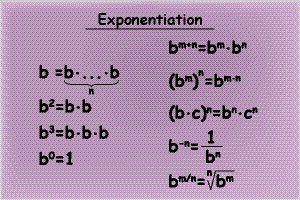# ALGEBRA: Simplifying Exponents with a Tutor in LONG BEACH

A Plus In Home Tutors helps Algebra students in Long Beach schools understand these rules in order to perform at their highest potential. Simplifying exponents does just that. It simplifies the expression so that you are dealing with a clear solution rather than a number “raised to the power.”Our A+ Algebra Tutors can teach you the rules used for simplifying those expressions.# ALGEBRA: Simplifying Exponents with a Tutor in LONG BEACH

Last Updated on September 30, 2020 by A Plus In-Home Tutors

When Algebra students start learning about exponents, there a few basic rules they must always remember to apply.

# A Plus In Home Tutors helps Algebra students in Long Beach schools understand these rules in order to perform at their highest potential.

Simplifying exponents does just that. It simplifies the expression so that you are dealing with a clear solution rather than a number “raised to the power.”

## Our A+ Algebra Tutors can teach you the rules used for simplifying those expressions.

Example:
Simplify (x³)(x⁴)
• To simplify this expression, you should remember a basic exponent rule.
• Rule: When multiplying two terms with the same base (x), you ADD the exponents.
• Therefore, (x³)(x⁴) = x³⁺⁴, which equals x⁷
Notice that the only time you can add the exponents is when the bases are the same. If they are different, you cannot combine them in Algebra.
What happens when you have to simplify an expression like this one:
Simplify (x²)⁴
• In the above expression, you cannot add 2 and 4 because “x squared” is inside the parentheses to the power outside the parentheses.
• Rule: Whenever you have an exponent expression that is raised to a power, you multiply the exponent and power.
• Therefore, (x²)⁴ = x⁸

### With the support of Firefly Tutors presented by A Plus In Home Tutors, you can practice simplifying these Algebra exponents by applying the correct rules.

The more you practice these, the easier Algebra becomes.
Algebra students in Long Beach high schools have learned that these rules do not work if the expression within the parentheses is a sum or a difference. Exponents, unlike multiplication, do not distribute over addition.
For example,
(3 + 4)² does not mean “three cubed” plus “four squared.” Do NOT distribute. First, find the sum of numbers inside the parentheses; then raise it to the 2nd power.
Many Algebra students make these mistakes because they rush through their work or try to guess without having learned the rules of Algebra.
A Plus In Home Tutors will make sure you are confident when simplifying exponents. Our Algebra tutors will take their time to show you step by step.
Sometimes in Algebra, there are exceptions to rules.
If you are dealing with an expression like (x-2)², you obviously cannot subtract 2 from x. Write it out instead. Remember that “squared” means “times itself,” so;
(x-2)² = (x-2)(x-2), now you can simplify using the FOIL Method
x² — 2x – 2x + 4
= x² — 4x + 4
Bonus Rule: Anything to the power zero is just “1”
These are some of the basic rules every Algebra student needs to know when simplifying exponents.
Whether you’re preparing for an upcoming Algebra test or need homework assistance, A Plus In Home Tutors is the right fit for you.

#### Visit us at www.myfireflytutors.com to find a great Algebra tutor near you!